# Go deh!

Mainly Tech projects on Python and Electronic Design Automation.

## Wednesday, August 26, 2020

### Python 3.9 graphlib review.

I had a look at the TopologicalSorter class and it seems to mix the graph algorithm in with a use case in a less than ideal way.

1. If given a graph of dependencies it will give one ordering that can satisfy the dependencies via its static_order() method only.
2. It supports one way to add parallelism.

I had written a Topological Sort task on Rosetta Code, a Python implementation, and had been both implementing and using T-sorts to  compile Electronics Design Automation libraries for over a decade, (maybe 20+ years).

Below, I make slight alterations to the RC toposort2 function to create toposort3, then compare it to the new graphlib.TopologicalSorter class.

## The Modules A, B, C, D example

They give the following input graph for T-sorting:

`graph = {"D": {"B", "C"},          "C": {"A"},          "B": {"A"}}`

Or more colloquially: D depends on, (or must come after),  both B and C; C depends on A and B depends on A.

The static_order ordering given is:

`('A', 'C', 'B', 'D')`

Now those dependencies have only a "looser" ordering of the set of nodes B and C. Nodes B and C can be swapped and it will still satisfy the dependencies. B and C can be implemented in parallel in fact.

My routine, instead of returning a iterator over individual items, instead iterates over sets of items.

1. All items in each set must be completed/ordered before all those of a preceding set from the iterator.
2. The items in each set may be ordered/completed in any way or completed in parallel.

My routines result iterator if turned into a tuple would yield:

({'A'}, {'B', 'C'}, {'D'})

If this were a calculated compilation order for Verilog and VHDL libraries then it clearly shows the opportunity for parallelism in compiling B and C and that a maximum of two libraries can be compiled in parallel.

The RosettaCode task graph shows even  more scope for parallelism.

Here is the task data run through the new module and my toposort function:

```from functools import reduce
from pprint import pprint as pp
from collections import defaultdict

from graphlib import TopologicalSorter

# LIBRARY     mapped_to     LIBRARY DEPENDENCIES
data = {
'des_system_lib':   set('std synopsys std_cell_lib des_system_lib dw02 dw01 ramlib ieee'.split()),
'dw01':             set('ieee dw01 dware gtech'.split()),
'dw02':             set('ieee dw02 dware'.split()),
'dw03':             set('std synopsys dware dw03 dw02 dw01 ieee gtech'.split()),
'dw04':             set('dw04 ieee dw01 dware gtech'.split()),
'dw05':             set('dw05 ieee dware'.split()),
'dw06':             set('dw06 ieee dware'.split()),
'dw07':             set('ieee dware'.split()),
'dware':            set('ieee dware'.split()),
'gtech':            set('ieee gtech'.split()),
'ramlib':           set('std ieee'.split()),
'std_cell_lib':     set('ieee std_cell_lib'.split()),
'synopsys':         set(),
}
for k, v in data.items():

# LIBRARY     mapped_to     LIBRARY PREDECESSORS
data_invert = defaultdict(set)
for lib, deps in data.items():
for dep in deps:

ts = TopologicalSorter(data)
graphlib_order = tuple(ts.static_order())

def toposort3(data):
extra_items_in_deps = reduce(set.union, data.values()) - set(data.keys())
data.update({item:set() for item in extra_items_in_deps})
while True:
ordered = set(item for item,dep in data.items() if not dep)
if not ordered:
break
yield ordered
data = {item: (dep - ordered) for item,dep in data.items()
if item not in ordered}
assert not data, "A cyclic dependency exists amongst %r" % data

print('Python 3.9 graphlib gives a topological ordering:')
pp(graphlib_order)

print('\nMy topological ordering of sets that must be in tuple order, but each item in an individual set can be executed in any order for that set, or in *parallel*:')
```

### Output:

```Python 3.9 graphlib gives a topological ordering:
('des_system_lib',
'dw03',
'dw07',
'dw06',
'dw04',
'dw05',
'ramlib',
'std_cell_lib',
'synopsys',
'dw02',
'dw01',
'std',
'dware',
'gtech',
'ieee')

My topological ordering of sets that must be in tuple order, but each item in an individual set can be executed in any order for that set, or in *parallel*:
({'synopsys', 'std', 'ieee'},
{'dware', 'ramlib', 'gtech', 'std_cell_lib'},
{'dw07', 'dw02', 'dw06', 'dw01', 'dw05'},
{'des_system_lib', 'dw04', 'dw03'})
```

From the output above, my routine doesn't hide what can be run in parallel, and encompasses every possible ordering of items, wheras the graphlib module only shows one ordering, and if the graphlib modules parallelism support is used, it is more difficult to get an idea of the opportunities for parallelism rather than just starting up another task.

I can see that I can use a maximum of five and a minimum of 3 tasks in parallel and that those parallel tasks can be executed in a minimum of four sequential steps.

So, That's why and how  I think graphlib could be better.

END.

## Tuesday, July 28, 2020

### More constrained random testing of set consolidation routines.

(Best read on more than a mobile phones screen)

In my last post on set consolidations I used random test data generation in comparing and contrasting three consolidation routines.

The test data generated was a list of thousands of sets of items with some duplication of items between sets.

I realise that whilst I extended the data to create hundreds of thousands of viable sets/set-like lists/"groups", individual sets all had less than twenty members.

I want to investigate performance when sets are much larger.

### Importing previous functions

In :
```#!pip install import_ipynb
```
In :
```import import_ipynb
import set_consolidation_speed
from set_consolidation_speed import consolidate, consolidate_np, consolidate_ramr, \
weighted_choice
```
```importing Jupyter notebook from set_consolidation_speed.ipynb
```

## Tall & skinny vs Short & fat

in the previous tests, no matter how many sets are generated, they all average around 5 elements in length over all sets and set lengths come from the same weighted random distribution. I can go "tall and skinny", but I also go "short and skinny"

This time I will adjust the random_data_gen function to have an extra `aspect` argument. The number of generated sets, `n`, will be divided by the aspect, whilst the length of sets to be randomly generated will be multiplied by `aspect`.

This way I keep the number of individual items of all the sets roughly constant when adjusting just the `aspect` term and keeping `n` constant. I should get some indication of how the consolidation functions cope with larger set sizes.
*(The term aspect is used as it affects [aspect ratio](https://en.wikipedia.org/wiki/Aspect_ratio)).*

In :
```import random
from pprint import pprint as pp

def rand_data_gen2(n=5, aspect=1, silent=False):
if not silent:
"Gen n//a sublists of randomised data with average set size multiplied by aspect"

# Need a pool of items to randomly select for each group
n_pool_items = int(2.5 * n)
pool_strings = [f'I{x}' for x in range(n_pool_items)]

# groups will be of ranodom but weighted size,
group_len_weights = ([(x, 5) for x in range(1, 6)] +    # lengths of 1..5 weights
[(x, 2) for x in range(6, 10)] +   # lengths of 6..9 weights
[(x, 1) for x in range(10, 16)])   # lengths of 10..15 weights
group_len_weights = [(ln, wt) for ln, wt in group_len_weights if ln <= n_pool_items]
group_len_gen = weighted_choice(group_len_weights)

data = [random.sample(pool_strings, next(group_len_gen) * aspect)
for _ in range(n // aspect)]

# stats
avg = sum(len(x) for x in data) / len(data)
if not silent:
print(f"""
GENERATED DATA STATS:
n:                                {n}
Aspect ratio:                     {aspect}
Row count:                        {len(data)}
Pool of random items:             {n_pool_items}
Average count of items per group: {avg}
""")

return data, avg
```
In :
```# This is just for repeatability and can be deleted
random.seed('RANDOM TEST DATA GENERATION')
```
In :
```if __name__ == '__main__':
for aspect_ratio in (1, 2, 4):
rand_data_gen2(2**15, aspect_ratio)
```
```          GENERATED DATA STATS:
n:                                32768
Aspect ratio:                     1
Row count:                        32768
Pool of random items:             81920
Average count of items per group: 5.380462646484375

GENERATED DATA STATS:
n:                                32768
Aspect ratio:                     2
Row count:                        16384
Pool of random items:             81920
Average count of items per group: 10.81591796875

GENERATED DATA STATS:
n:                                32768
Aspect ratio:                     4
Row count:                        8192
Pool of random items:             81920
Average count of items per group: 21.30322265625

```

Note how the average group size, (approximately), doubles as the row count successively halves.

## Timings

I'll use pandas for its data formatting

In :
```import timeit
import datetime
import pandas as pd
import copy

def runtime2(methods, random_data):
"Time the methods acting on the data"
rep = 2
times = []
runtime2.random_data = random_data
for method in methods:
name = method.__name__
cmd = f"{name}(ddd)"
setup = (f"from __main__ import copy, {name}, runtime2\n"
"ddd = copy.deepcopy(runtime2.random_data)")
t = timeit.Timer(cmd, setup
).timeit(number=rep) / rep
times.append(datetime.timedelta(seconds=t))
return times

def method_compare(methods, rows=[128, 256], aspects=[1, 2, 4]):
"Compare timings of methods with data generated from specified row counts and aspect ratios"
results = {}
for key in 'n, aspect, rows, avg_set_len:'.split():
results[key] = []
method_names = [m.__name__ for m in methods]
for key in method_names:
results[key] = []

for n in rows:
for aspect in aspects:
data, avg_set_len = rand_data_gen2(n, aspect, silent=True)
times = runtime2(methods, data)
for key, val in zip('n, aspect, rows, avg_set_len:'.split(),
(n, aspect, n // aspect, avg_set_len)):
results[key].append(val)
for key, val in zip(method_names, times):
results[key].append(val)
return pd.DataFrame(results)
```

## Trial timings on all methods

In :
```methods = [consolidate, consolidate_ramr, consolidate_np]
rows = [2**4, 2**8]
aspects = [1, 2]

results = method_compare(methods, rows, aspects)
results
```
Out:
n,aspect,rows,avg_set_len:consolidateconsolidate_ramrconsolidate_np
0161165.31250000:00:00.00004800:00:00.00006500:00:00.003666
116287.50000000:00:00.00002100:00:00.00003400:00:00.000657
225612565.48437500:00:00.00213500:00:00.00078900:00:06.598615
3256212810.96875000:00:00.00083400:00:00.00067000:00:01.828410

## Drop the numpy solution

It is obviousely extremely slow compared to the others so do a larger run without it

In :
```methods = [consolidate, consolidate_ramr]
rows = [2**10, 2**12]
aspects = [2**x for x in range(7)]

results2 = method_compare(methods, rows, aspects)
results2
```
Out:
n,aspect,rows,avg_set_len:consolidateconsolidate_ramr
01024110245.35156200:00:00.02881100:00:00.003646
11024251210.48046900:00:00.01066900:00:00.003078
21024425621.23437500:00:00.00761000:00:00.002711
31024812842.68750000:00:00.00437900:00:00.002209
41024166483.25000000:00:00.00247800:00:00.001867
510243232222.00000000:00:00.00183400:00:00.002240
610246416392.00000000:00:00.00110900:00:00.002076
74096140965.54467800:00:00.50679400:00:00.014725
840962204810.45410200:00:00.23905200:00:00.012017
940964102420.72265600:00:00.13112800:00:00.010961
104096851242.40625000:00:00.07120300:00:00.008084
1140961625691.68750000:00:00.03778300:00:00.008381
12409632128178.25000000:00:00.02233600:00:00.009287
1340966464352.00000000:00:00.01113300:00:00.014599

## Graphed runtimes

In :
```methods = [consolidate, consolidate_ramr]
rows = [2**x for x in range(10, 14)]
aspects = [1, 4, 16, 64, 256]
for n in rows:
res = method_compare(methods, [n], aspects)
res.plot.line(y=['consolidate', 'consolidate_ramr'], x='avg_set_len:', title=f'RUNTIME. n={n} for all aspect changes')
```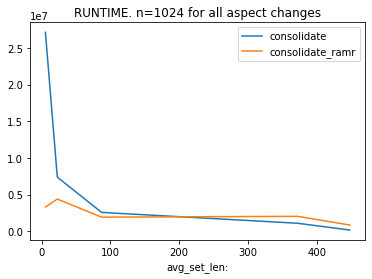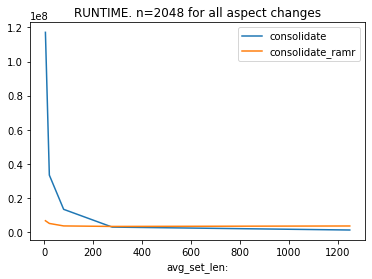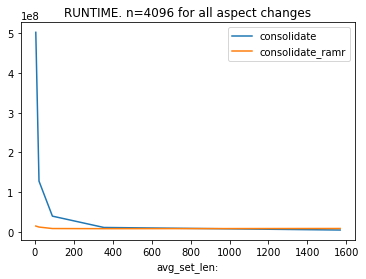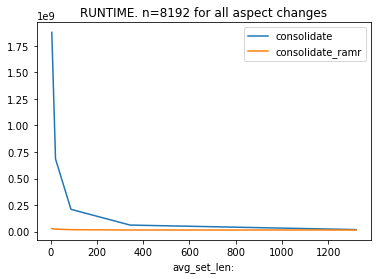In :
```display(res)
```
n,aspect,rows,avg_set_len:consolidateconsolidate_ramr
08192181925.38696300:00:01.87630800:00:00.029510
181924204821.51953100:00:00.68439000:00:00.024539
281921651288.18750000:00:00.21060300:00:00.019065
3819264128343.50000000:00:00.06243200:00:00.015940
48192256321320.00000000:00:00.01985400:00:00.016067

As the average set length rises in each graph, the number of sets to compare in the `consolidate` Python sets method comes down and its run times comes down to that of the `consolidate_ramr` method but the `consolidate` method is clearly slower. The Python sets based `consolidate` clearly shows the cost associated with more sets to process.

The runtimes for `consolidate_ramr` stay low, and fairly constant as the aspect ratio is changed (but the overall number of items in sets stays roughly the same).

### How run times change with the number of sets

remembering that the number of items in the sets stays roughly constant but the number of sets changes from `n` x sets of size `s` to `n/256` sets of size `s*256`

In :
```# consolidate RUNTIME CHANGES OVER 1-to-256 ASPECT CHANGE
res['consolidate'].value / res['consolidate'].value
```
Out:
`2.7415771709113224`
In :
```# consolidate_ramr RUNTIME CHANGES OVER 1-to-256 ASPECT CHANGE
res['consolidate_ramr'].value / res['consolidate_ramr'].value
```
Out:
`1.2025754920738416`

## root-and-merge-roots with bigdata

Lets drop the slower `consolidate` routine and investigate the ramr routine with bigger data

In :
```methods = [consolidate_ramr]
rows = [2**x for x in [16, 20]]
aspects = [1, 4, 16, 64, 256]
for n in rows:
res = method_compare(methods, [n], aspects)
res.plot.line(y=['consolidate_ramr'], x='avg_set_len:', title=f'RUNTIME. n={n} for all aspect changes')
display(res)

```
n,aspect,rows,avg_set_len:consolidate_ramr
0655361655365.34909100:00:00.365121
16553641638421.60473600:00:00.289843
26553616409687.07421900:00:00.247693
365536641024339.43750000:00:00.208501
4655362562561269.00000000:00:00.194705
n,aspect,rows,avg_set_len:consolidate_ramr
01048576110485765.38434000:00:09.082441
11048576426214421.55221600:00:06.862761
21048576166553686.58105500:00:05.594431
310485766416384344.22265600:00:05.245034
4104857625640961391.87500000:00:04.957202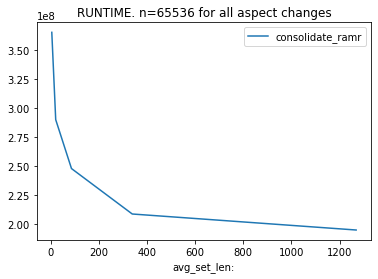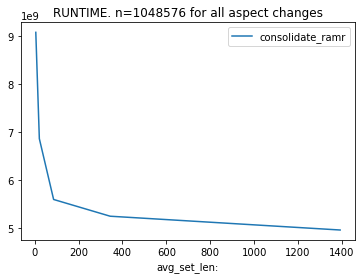With only the one method being graphed, we get a scale change that shows that the `consolidate_ramr` method also shows longer run times when processing more (but smaller) sets.

In :
```# consolidate_ramr RUNTIME CHANGES OVER 1-to-256 ASPECT CHANGE
res['consolidate_ramr'].value / res['consolidate_ramr'].value
```
Out:
`1.323438336261455`
In [ ]:
` `